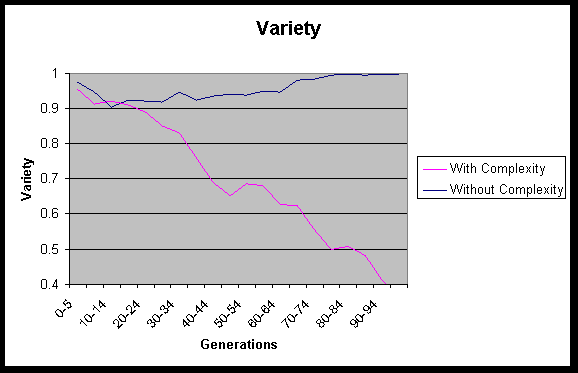Population Variety (with complexity vs. without complexity)

This graph is one of the most intriguing of all of the results, demonstrating the dramatic effect of introducing complexity as a factor in fitness. In the test that used complexity as part of fitness, the relative penalty gained by a complex individual was very small. (The final formula was to add complexity/50 to the fitness measure. The fitness measures varied between 70 and 200, while complexity ran from 1 to 100--I would guess that most individuals saw a roughly 1% increase in their fitness measures due to complexity). However, the average fitness and the average complexity graphs demonstrate the dramatic effect that this had on the complexity and fitness of the population over time. Further, as this graph demonstrates, it effectively limited the population of the test using complexity to numerous occurances of a small number of individuals, while the test that did not use complexity was composed of almost entirely unique individuals, allowing the search space to continue to expand.
William Bauder
wjb14@columbia.edu